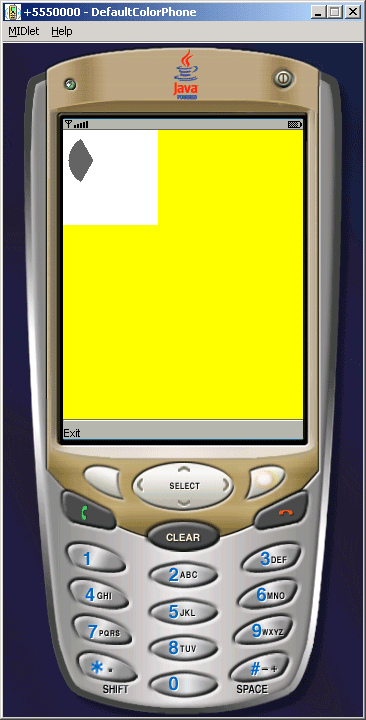Tutorials

# Co-ordinates MIDlet Example

In this example the CoordinatesCanvas class extends the Canvas class to draw the image and fill color as given below in the figure.

In this example the CoordinatesCanvas class extends the Canvas class to draw the image and fill color as given below in the figure.

# Co-Ordinates MIDlet Example

In this example the CoordinatesCanvas class extends the Canvas class  to draw the image and fill color as given below in the figure. The following methods is used to draw the image:

• image = Image.createImage(100, 100);
• Graphics g = image.getGraphics();
• g.setColor(110,110,110);
• g.fillArc(10, 10, 50, 50, 120, 120);

In the paint(Graphics g) function the following methods is used to color the image:

• g.setColor(255,255,0);
• g.fillRect(0, 0, getWidth(), getHeight());
• g.translate(45, 45);
• g.drawImage(image, 0, 0, Graphics.VCENTER | Graphics.HCENTER);

Simply the commandAction function is used to exit the current window and the application is as follows:Source Code of Coordinates.java

 ```import javax.microedition.midlet.*; import javax.microedition.lcdui.*; public class Coordinates extends MIDlet{   private Display  display;    private CoordinatesCanvas canvas;       public Coordinates (){   display = Display.getDisplay(this);   canvas  = new CoordinatesCanvas();   }   protected void startApp(){   display.setCurrent( canvas );   }   protected void pauseApp(){ }   protected void destroyApp( boolean unconditional ){   notifyDestroyed();   }   class CoordinatesCanvas extends Canvas implements CommandListener{   private Command exit;     private Image image;      public CoordinatesCanvas(){   exit = new Command("Exit", Command.EXIT, 1);   addCommand(exit);   setCommandListener(this);   try{   image = Image.createImage(100, 100);   Graphics g = image.getGraphics();   g.setColor(110,110,110);   g.fillArc(10, 10, 50, 50, 120, 120);     }catch (Exception error){   Alert alert = new Alert("Failure", "Creating Image", null, null);   alert.setTimeout(Alert.FOREVER);   display.setCurrent(alert);   }     }    protected void paint(Graphics g){   if (image != null){   g.setColor(255,255,0);   g.fillRect(0, 0, getWidth(), getHeight());   g.translate(45, 45);   g.drawImage(image, 0, 0, Graphics.VCENTER | Graphics.HCENTER);     }   }   public void commandAction(Command command, Displayable display){   String label = command.getLabel();   if (label.equals("Exit")){   destroyApp(true);   }   }   } }```